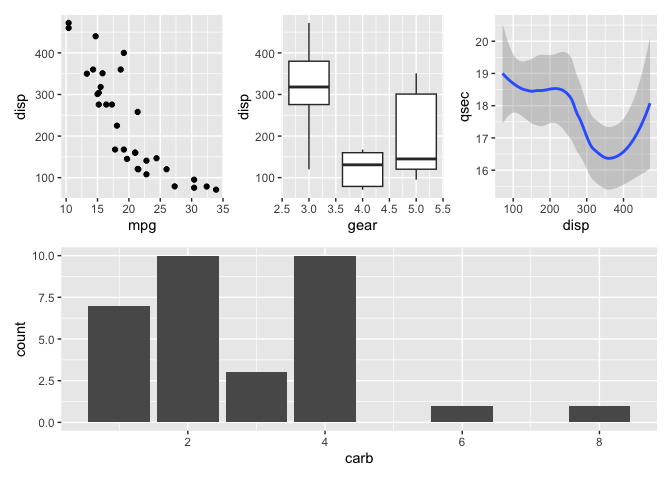The goal of patchwork is to make it ridiculously simple to combine separate ggplots into the same graphic. As such it tries to solve the same problem as gridExtra::grid.arrange() and cowplot::plot_grid but using an API that incites exploration and iteration, and scales to arbitrily complex layouts.

## Installation

You can install patchwork from CRAN using install.packages('patchwork'). Alternatively you can grab the development version from github using devtools:

# install.packages("devtools")
devtools::install_github("thomasp85/patchwork")

## Basic example

The usage of patchwork is simple: just add plots together!

library(ggplot2)
library(patchwork)

p1 <- ggplot(mtcars) + geom_point(aes(mpg, disp))
p2 <- ggplot(mtcars) + geom_boxplot(aes(gear, disp, group = gear))

p1 + p2patchwork provides rich support for arbitrarily complex layouts with full alignment. As an example, check out this very readable code for nesting three plots on top of a third:

p3 <- ggplot(mtcars) + geom_smooth(aes(disp, qsec))
p4 <- ggplot(mtcars) + geom_bar(aes(carb))

(p1 | p2 | p3) /
p4